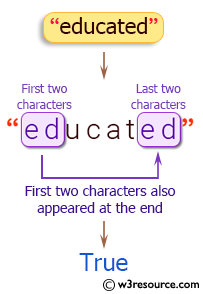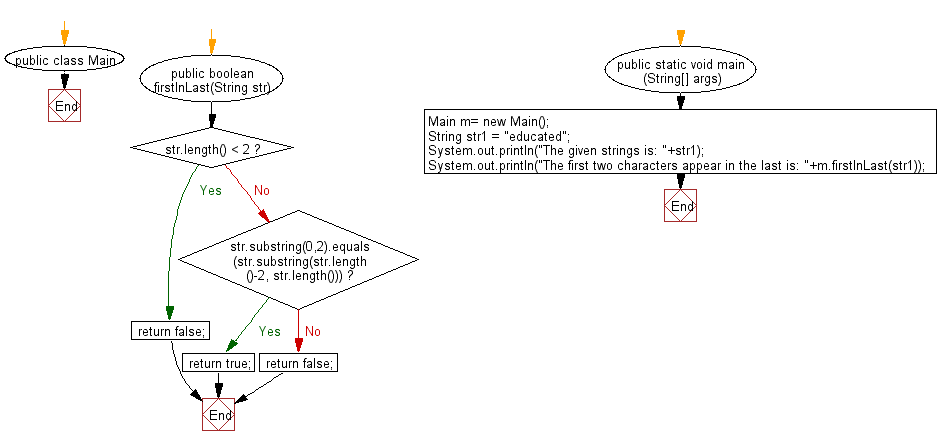﻿ Java exercises: Check whether the first two characters present at the end of a given string - w3resource# Java String Exercises: Check whether the first two characters present at the end of a given string

## Java String: Exercise-63 with Solution

Write a Java program to check whether the first two characters present at the end of a given string.

Sample Solution:

Java Code:

``````import java.util.*;
public class Main
{
public boolean firstInLast(String str)
{
if (str.length() < 2)
return false;
else if (str.substring(0,2).equals(str.substring(str.length()-2, str.length())))
return true;
else
return false;
}
public static void main (String[] args)
{
Main m= new Main();
String str1 =  "educated";

System.out.println("The given strings is: "+str1);
System.out.println("The first two characters appear in the last is: "+m.firstInLast(str1));
}
}
```
```

Sample Output:

```The given strings is: educated
The first two characters appear in the last is: true
```

Pictorial Presentation:Flowchart:Java Code Editor:

Improve this sample solution and post your code through Disqus

What is the difficulty level of this exercise?

﻿

## Java: Tips of the Day

Parsing dates:

```import java.io.*;
import java.util.*;
import java.text.*;

String s = "2001/09/23 14:39";

SimpleDateFormat formatter = new SimpleDateFormat ("yyyy/MM/dd H:mm");
Date d = formatter.parse(s, new ParsePosition(0));
```